MCAT Physical : Magnetism and Electromagnetism

Example Questions

← Previous 1

Example Question #1 : Magnetism And Electromagnetism

You look at a circular loop of wire such that the plane of the loop is perpendicular to your line of vision. The loop has a constant current that is running through it clockwise. What will happen if a magnetic field were to be activated that is pointing in your direction?

The current in the loop will continue clockwise but will increase

The current in the loop will begin to move counterclockwise and slowly increase

The current in the loop will begin to move counterclockwise at the same magnitude of current as before

The current in the loop will begin to move counterclockwise but slowly decrease

The current in the loop will continue clockwise but will decrease

The current in the loop will continue clockwise but will increase

Explanation:

This question works with the concept of induction. Simply put, the current in a wire will adjust such as to oppose a change in magnetic field. The loop originally has a magnetic field pointing away from the observer. Therefore, with the external magnetic field suddenly activated in the opposite direction (towards the observer), the current in the loop will act to counteract this change and increase while remaining clockwise.

Example Question #2 : Magnetism And Electromagnetism

An electron moves at 85km/s to the right along the plane of the page, while a uniform magnetic field points into the page. In what direction does the force act on the moving electron?

To the right

Upward along the plane of the page

Downward along the plane of the page

Into the page at an angle

Out of the page

Upward along the plane of the page

Explanation:

This question requires knowlegde of the right-hand rule. Point the fingers of your right hand in the direction of the electron's velocity (to the right). Point your thumb in the direction of the magnetic field (into the page). Your palm should be facing in the direction of the force on a positive particle. However, electrons are negative, so this direction must be reversed, meaning that the direction of the force is upward along the plane of the page.

Example Question #3 : Magnetism And Electromagnetism

You look at a circular loop of wire such that the plane of the loop is perpendicular to your line of vision. In what direction must a current be going through the loop in order for a magnetic field to be produced in your direction.

Counterclockwise

Clockwise and slowly increasing

Clockwise

Clockwise but slowly decreasing

The direction of the current does not matter

Counterclockwise

Explanation:

This is an application of the right hand rule for magnetic fields produced by current carrying loops. To use the right hand rule, put your right thumb in the direction of the current, and the direction of the magnetic field is the same as the way your other four fingers wrap as you close your fist. The answers which include increasing or decreasing of a current hint at the concept of induction and are incorrect.

Example Question #1 : Magnetism And Electromagnetism

Which of the following influences the emf produced in a wire loop that is rotating in a magnetic field?

The resistance of the loop

The shape of the loop

The size of the loop

The material of which the loop is made

More than one of the other options is correct

The size of the loop

Explanation:

Recall that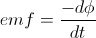, where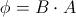. The shape, material characteristics, and resistance do not appear in this equation. So only the size (area) of the loop influences the emf.

Example Question #5 : Magnetism And Electromagnetism

Which of the following best describes magnetic field lines?

They can only cross when multiple magnets are present

More than one of the other options is correct

They always begin at a north pole and terminate at a south pole

They show both the relative strength and direction of the magnetic field

They show the direction in which a positive charge will experience a force

They show both the relative strength and direction of the magnetic field

Explanation:

First, magnetic field lines, like electric field lines, can never cross. Also, unlike electric field lines, magnetic field lines are continuous—they do not have starting or ending points. Next, the force experienced by a charge in a magnetic field depends on the charge's velocity direction, not just the magnetic field. So the only remaining choice is that magnetic field lines show both the relative strength and direction of the field.

Example Question #6 : Magnetism And Electromagnetism

A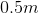current-carrying wire is placed in a uniform magnetic field oriented perpendicularly to the wire. The length of the wire is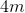and the magnitude of the magnetic field is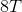. What is the force on the wire?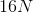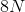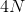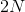Explanation:

We use the formula for force on a wire in a magnetic field: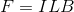Where= force,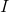= current,= length of wire, and= magnetic mield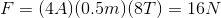Example Question #7 : Magnetism And Electromagnetism

A scientist builds a particle accelerator. Which of the following would not help the scientist increase the speed of a particle in the accelerator?

Increasing the charge of the particles

Increasing the radius that the particles spin around

Increasing the mass of the particles in the accelerator

Increasing the strength of the magnetic field

Decreasing the temperature of the particle accelerator

Increasing the mass of the particles in the accelerator

Explanation:

A charged particle in a particle accelerator moves in uniform circular motion. The equation for centripetal force caused by uniform circular motion is: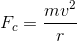The equation for magnetic force is: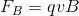Set the equations equal to each other, and solve for velocity.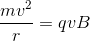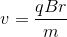The only answer choice that does not correspond to an increase in velocity is increasing the mass of the particles in the accelerator.

Example Question #1 : Magnetism And Electromagnetism

An electron is traveling at constant velocity directed to the the left through a magnetic field directed into the page as shown below.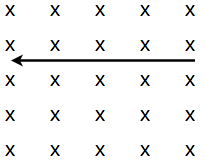Which way would the force vector on the electron due to the magnetic field be directed?

Towards the bottom of the page

Towards the right of the page

Towards the top of the page

Out of the page

Towards the top of the page

Explanation:

When finding the direction of the force on a POSITIVELY charged particle due to a magnetic field, we can use the right hand rule. Holding your thumb perpendicular to the rest of your fingers (as if motioning to stop), orient your fingers parallel to the lines of the magnetic field, and your thumb with the velocity vector of the charge. For a positive charge, the force would be oriented directly out of the palm; HOWEVER, the charge of an electron is negative and therefore the force will be applied in the opposite direction. This would mean that the force would be coming directly out of the backside of your hand (in this case, towards the top of the page) using the right hand rule.

Example Question #9 : Magnetism And Electromagnetism

A charged particle enters a uniform magnetic field, with velocity v perpendicular to the field, and moves in a circular path of radius R. If the particle's mass were doubled, the radius would become __________.

R/2

4R

R/4

R

2R

2R

Explanation:

A charged particle's motion in a uniform magnetic field is described by R = mv/qB, so the radius of the particle's path is proportional to its mass. Thus if mass is doubled, radius is also doubled.

Example Question #1 : Magnetic Forces And Energy

An electron is moving at a constant velocity due east. It travels from a region of zero magnetic field into a uniform magnetic field of nonzero magnitude and unknown direction. Which of the following could not describe the electron's motion after entering the field?

Its path begins curving to the north

It travels in a helical path

Its path begins curving to the south

It continues traveling east, but at a greater velocity

It continues traveling east at the same velocity

It continues traveling east, but at a greater velocity

Explanation:

A magnetic force can accelerate a charged particle by changing the direction of its velocity, but cannot change the magnitude of velocity.

If the magnetic field has any components perpendicular to the particle's initial velocity, the particle will experience a force according to the equation: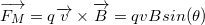This force is generated perpendicular to the particle's path and will cause a change in the direction of the particle's velocity. If the magnetic field is parallel to the initial velocity, the particle will experience no magnetic force, and its path will remain unchanged. It is not possible for the particle to accelerate in the magnetic field without changing direction.

← Previous 1